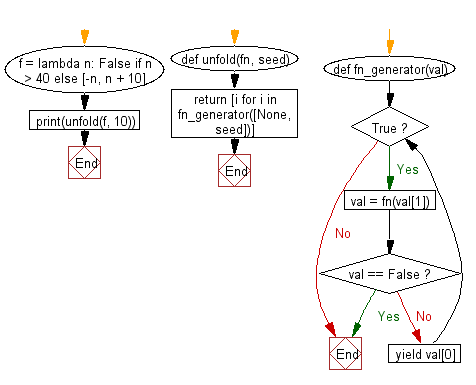﻿ Python: Build a list, using an iterator function and an initial seed value - w3resource# Python: Builds a list, using an iterator function and an initial seed value

## Python List: Exercise - 219 with Solution

Write a Python program to build a list, using an iterator function and an initial seed value.

• The iterator function accepts one argument (seed) and must always return a list with two elements ([value, nextSeed]) or False to terminate.
• Use a generator function, fn_generator, that uses a while loop to call the iterator function and yield the value until it returns False.
• Use a list comprehension to return the list that is produced by the generator, using the iterator function.

Sample Solution:

Python Code:

``````def unfold(fn, seed):
def fn_generator(val):
while True:
val = fn(val)
if val == False: break
yield val
return [i for i in fn_generator([None, seed])]
f = lambda n: False if n > 40 else [-n, n + 10]
print(unfold(f, 10))
```
```

Sample Output:

```[-10, -20, -30, -40]
```

Flowchart:## Visualize Python code execution:

The following tool visualize what the computer is doing step-by-step as it executes the said program:

Python Code Editor:

Have another way to solve this solution? Contribute your code (and comments) through Disqus.

What is the difficulty level of this exercise?

Test your Python skills with w3resource's quiz

﻿

## Python: Tips of the Day

Floor Division:

When we speak of division we normally mean (/) float division operator, this will give a precise result in float format with decimals.

For a rounded integer result there is (//) floor division operator in Python. Floor division will only give integer results that are round numbers.

```print(1000 // 300)
print(1000 / 300)```

Output:

```3
3.3333333333333335```